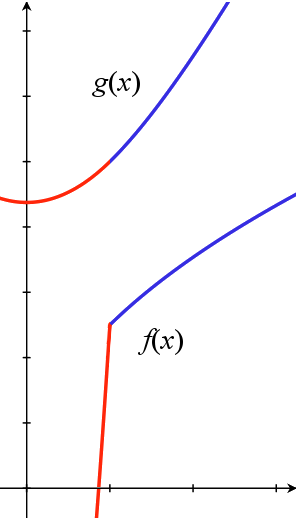Difference between revisions of "009A Sample Final A, Problem 3"Both functions with constants chosen to provide continuity.

3. (Version I) Consider the following function:  $f(x)={\begin{cases}{\sqrt {x}},&{\mbox{if }}x\geq 1,\\4x^{2}+C,&{\mbox{if }}x<1.\end{cases}}$(a) Find a value of  $C$which makes $f$continuous at $x=1.$(b) With your choice of  $C$, is $f$differentiable at $x=1$?  Use the definition of the derivative to motivate your answer.

3. (Version II) Consider the following function:  $g(x)={\begin{cases}{\sqrt {x^{2}+3}},&\quad {\mbox{if }}x\geq 1\\{\frac {1}{4}}x^{2}+C,&\quad {\mbox{if }}x<1.\end{cases}}$(a) Find a value of  $C$which makes $f$continuous at $x=1.$(b) With your choice of  $C$, is $f$differentiable at $x=1$?  Use the definition of the derivative to motivate your answer.

Foundations:
A function $f$is continuous at a point $x_{0}$if
$\lim _{x\rightarrow x_{_{0}}}f(x)=f\left(x_{0}\right).$This can be viewed as saying the left and right hand limits exist, and are equal to the value of $f$at $x_{0}$. For problems like these, where we are trying to find a particular value for  $C$, we can just set the two descriptions of the function to be equal at the value where the definition of $f$changes.
When we speak of differentiability at such a transition point, being "motivated by the definition of the derivative" really means acknowledging that the derivative is a limit, and for a limit to exist it must agree from the left and the right. This means we must show the derivatives agree for both the descriptions of $f$at the transition point.

Solution:

Version I:
(a) For continuity, we evaluate both rules for the function at the transition point $x=1$, set the results equal, and then solve for $C$. Since we want
$f(1)\,=\,1\,=\,4\cdot 1^{2}+C,$we can set $C=-3$, and the function will be continuous (the left and right hand limits agree, and equal the function's value at the point $x=1$).

(b) To test differentiability, we note that for $x>1$,

$f'(x)={\frac {1}{2{\sqrt {x}}}},$while for $x<1$,
$f'(x)=8x.$Thus
$\lim _{x\rightarrow 1^{+}}f'(x)\,=\,{\frac {1}{2{\sqrt {1}}}}\,=\,{\frac {1}{2}},$but
$\lim _{x\rightarrow 1^{-}}f'(x)\,=\,8\cdot 1\,=\,8.$Since the left and right hand limit do not agree, the derivative does not exist at the point $x=1$.
Version II:
(a) Like Version I, we begin by setting the two functions equal. We want
$f(1)\,=\,{\sqrt {1^{2}+3}}\,=\,2\,=\,{\frac {\,\,1^{2}}{4}}+C,$so $C=7/4$makes the function continuous.
(b) We again consider the derivative from each side of 1. For $x>1$,
$f'(x)={\frac {1}{2{\sqrt {x^{2}+3}}}}\cdot 2x\,=\,{\frac {x}{\sqrt {x^{2}+3}}},$while for $x<1$,
$f'(x)\,=\,{\frac {x}{2}}.$Thus
$\lim _{x\rightarrow 1^{+}}f'(x)\,=\,{\frac {1}{\sqrt {1^{2}+3}}}\,=\,{\frac {1}{2}},$and
$\lim _{x\rightarrow 1^{-}}f'(x)\,=\,{\frac {1}{2}}.$Since the left and right hand limit do agree, the limit (which is the derivative) does exist at the point $x=1$, and $g(x)$is differentiable at the required point.